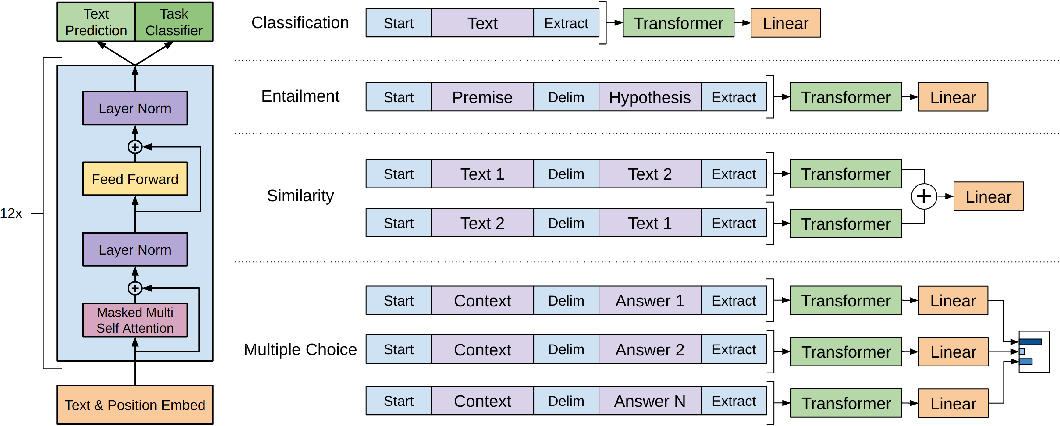# GPT: Generative Pre-Training

Improving Language Understanding by Generative Pre-Training

## Takeaways

• Large gains can be realized by generative pre-training + discriminative fine-tuning on each specific task.
• Task-aware input transformations during fine-tuning achieve effective transfer while requiring minimal changes to the model architecture.

## Introduction

Learning from unlabeled data

• Large labeled datasets are unavailable in many domains that suffer from a dearth of annotated resources.
• Large unlabeled text corpora are abundant
• Linguistic information from unlabeled data provides a valuable alternative to gathering more annotation.
• Even in cases where considerable supervision is available, learning good representations in an unsupervised fashion can provide a significant performance boost.

Challenges

• What type of optimization objectives are most effective at learning text representations that are useful for transfer?
• No consensus on the most effective way to transfer these learned representations to the target task

This work: unsupervised pre-training + supervised fine-tuning

## MethodsLeft: Transformer architecture and training objectives. Right: input transformations for fine-tuning on different tasks.

### Unsupervised Pre-Training

#### Objective

Standard language modeling:

$L_1(\mathcal{U}) = \sum_i\log P(u_i|u_{i-k},\dots, u_{i-1}; \Theta)$

where $$\mathcal{U}=\{u_1,\dots,u_n\}$$ is an unlabeled corpus of tokens, $$k$$ is the size of the context window, and $$\Theta$$ is the parameters of network.

#### Model

Multi-layer Transformer decoder.

#### Dataset

The BooksCorpus dataset is used for pre-training, which contains over 7,000 unique unpublished books from a variety of genres.

### Supervised Fine-Tuning

After pre-training the model, adapt the parameters to the supervised target task.

Let $$\mathcal{C}$$ be a labeled dataset where each instance consists of a sequence of input tokens, $$x^1,\dots,x^m$$, and a label $$y$$. The inputs are passed through the pre-trained model to obtain the final transformer block’s activation $$h_l^m$$, which is then fed into an added linear output layer with parameters $$W_y$$ to predict $$y$$:

$P(y|x^1,\dots, x^m)=\texttt{softmax}(h_l^m W_y).$

Use log-loss:

$L_2(\mathcal{C}) = \sum_{(x,y)}\log P(y|x^1,\dots, x^m)$

Including language modeling as an auxiliary objective to the fine-tuning, in order to

• improve generalization of the supervised model,
• accelerate convergence.

Total loss:

$L_3(\mathcal{C}) = L_2(\mathcal{C}) + \lambda L_1(\mathcal{C}).$

For some tasks, like text classification, the inputs can be used as is.

Since tje pre-trained model was trained on contiguous sequences of text, some modifications are required for tasks with different formats of inputs, e.g., sentence pairs, triplets of document, question, and answers.

All transformations include adding randomly initialized start and end tokens (<s>, <e>).

#### Textual Entailment

concatenate the premise $$p$$ and hypothesis $$h$$ token sequences, with a delimiter token (\$) in between.

#### Similarity

There is no inherent ordering of the two sentences being compared. Modify the input sequence to contain both possible sentence orderings (with a delimiter in between) and process each independently to produce two sequence representations which are added element-wise before being fed into the linear output layer.

#### Question Answering and Commonsense Reasoning

Concatenate the document context and question with each possible answer, adding a delimiter token in between to get $$[z; q; \; a_k]$$. Each of these sequences is processed independently with the model and then normalized via a softmax layer to produce an output distribution over possible answers.

## Experiments

### Results of Fine-Tuning

Overall, GPT achieves new SOTA results in 9 out of the 12 datasets, outperforming ensembles in many cases. Results also indicate that GPT works well across datasets of different sizes.

### Impact of Number of Layers Transferred

Transferring embeddings improves performance and each transformer layer provides further benefits. This indicates that each layer in the pre-trained model contains useful functionality for solving target tasks.

### Zero-shot Behaviors

#### Hypothesis

The underlying generative model learns to perform many of the tasks in order to improve its language modeling capability and the more structured attentional memory of the transformer assists in transfer compared to LSTMs.

#### Test

Evaluate the zero-shot performance over the course of pre-training.

The zero-shot performance is stable and steadily increases over training suggesting that generative pretraining supports the learning of a wide variety of task-relevant functionality. Also, the LSTM exhibits higher variance in its zero-shot performance suggesting that the inductive bias of the Transformer architecture assists in transfer.